# 博客作业05-指针

| 这个作业属于哪个班级 | C语言--网络2011/2012 |
| ---- | ---- | ---- |
| 这个作业的地址 | (https://edu.cnblogs.com/campus/jmu/2020C/homework/11632) |
| 这个作业的目标 | 学习指针相关内容。 |

# 0展示pta总分# 1本章学习总结

## 1.1指针定义、指针相关运算、指针做函数参数

• 定义
指针是一个用来指示一个内存地址的计算机语言的变量或中央处理器(CPU)中的寄存器(Register)。指针一般指向一个函数或一个变量。在使用一个指针时，一个程序既可以直接使用这个指针所储存的内存地址，又可以使用这个地址里储存的函数的值。指针变量类型不是指指针变量本身的类型，而是指它所指向的变量的数据类型。指针变量也要先赋值再使用，指针变量被赋值的值应该是地址。
定义指针变量：类型名 *指针变量名

• 相关运算
对指针变量p赋值：1、p=&i;p=0;p=NULL;p=(int)123;同类型指针才能互相赋值。
一个指针p所指向变量的值加1( p=p+1、++p和（P)++ )指针变量相加无实际意义
两个指针变量之差是两个指针之间的元素个数。

• 指针做函数参数
使用指针形参

#include <stdio.h>
void found(int* num);
nt main()
{
int count=0;
found(count);
return 0;
}
void found(int* num)
{
(*num)++;
}


## 1.3指针做函数返回值

char* (char* str1,char* str2)
{
if(strcmp(str1,str2)>0)
return str1;
else
return str2;
}


## 1.4动态内存分配

• 解释
在程序执行的过程中动态地分配或者回收存储空间的分配内存的方法。动态内存分配不象数组等静态内存分配方法那样需要预先分配存储空间，而是由系统根据程序的需要即时分配，且分配的大小就是程序要求的大小。
• 动态存分配原因
当程序运行到需要一个动态分配的变量或对象时，必须向系统申请取得堆中的一块所需大小的存贮空间，用于存贮该变量或对象。当不再使用该变量或对象时，也就是它的生命结束时，要显式释放它所占用的存贮空间，这样系统就能对该堆空间进行再次分配，做到重复使用有限的资源
• 堆区
由程序员调用malloc()函数来主动申请的，需使用free()函数来释放内存，若申请了堆区内存，之后忘记释放内存，很容易造成内存泄漏。
通常定义变量（或对象），编译器在编译时都可以根据该变量（或对象）的类型知道所需内存空间的大小，从而系统在适当的时候为他们分配确定的存储空间。这种内存分配称为静态存储分配；有些操作对象只在程序运行时才能确定，这样编译时就无法为他们预定存储空间，只能在程序运行时，系统根据运行时的要求进行内存分配，这种方法称为动态存储分配。所有动态存储分配都在堆区中进行。
• 栈区
存放函数内的局部变量，形参和函数返回值。栈区之中的数据的作用范围过了之后，系统就会回收自动管理栈区的内存(分配内存 , 回收内存),不需要开发人员来手动管理。
• 区别
| 堆区的栈区的区别 | 堆区 | 栈区 |
| ---- | ---- | ---- |
| 申请方式 | 由程序员自己申请，并指明大小 | 由系统自动分配，函数结束时释放 |
| 申请大小的限制 | 看主机是多少位的 | 1或者2M，可以自己改，但是最大不超过8M |
| 申请效率 | 一般速度较慢 | 速度较快 |
| 存储内容 | 一般在堆的头部用一个字节存放堆的大小，堆中的具体内容由程序员安排 | 函数调用（静态变量不入栈） |
| 管理方式不同 | 链表 | 数据结构中的栈 |
| 生长方向 | 低到高 | 高到低 |
不知道为什么表格出不来
• 动态内存分配相关函数及用法
** colloc **
void* colloc(unsigned int n,unsigned int size);分配n个连续存储单元（每个单元包含size字节）。返回值：成功：分配单元首地址，失败：NULL
** malloc **
void* maolloc(unsigned int size);分配size个字节的存储单元块。返回值：成功：分配单元首地址，失败：NULL
** free **
void free(void p);释放p所致存储单元块（必须是由动态内存分配函数一次性分配的全部单元），返回值：无
** realloc **
void
realloc(void*p,unsigned int size);将p所指的已分配存储单元块的大小改为size,返回值：成功：单元快首地址，失败：NULL
colloc() malloc()函数需强制类型转换成所需单元类型的指针。
• 为多个字符串做动态内存分配
  char *color, str;
scanf("%s", str);
color[n] = (char *) malloc( sizeof(char) * ( strlen(str) + 1 ));


## 1.6 二级指针

  int a=10;
int* p=&a;
int** pp=&p;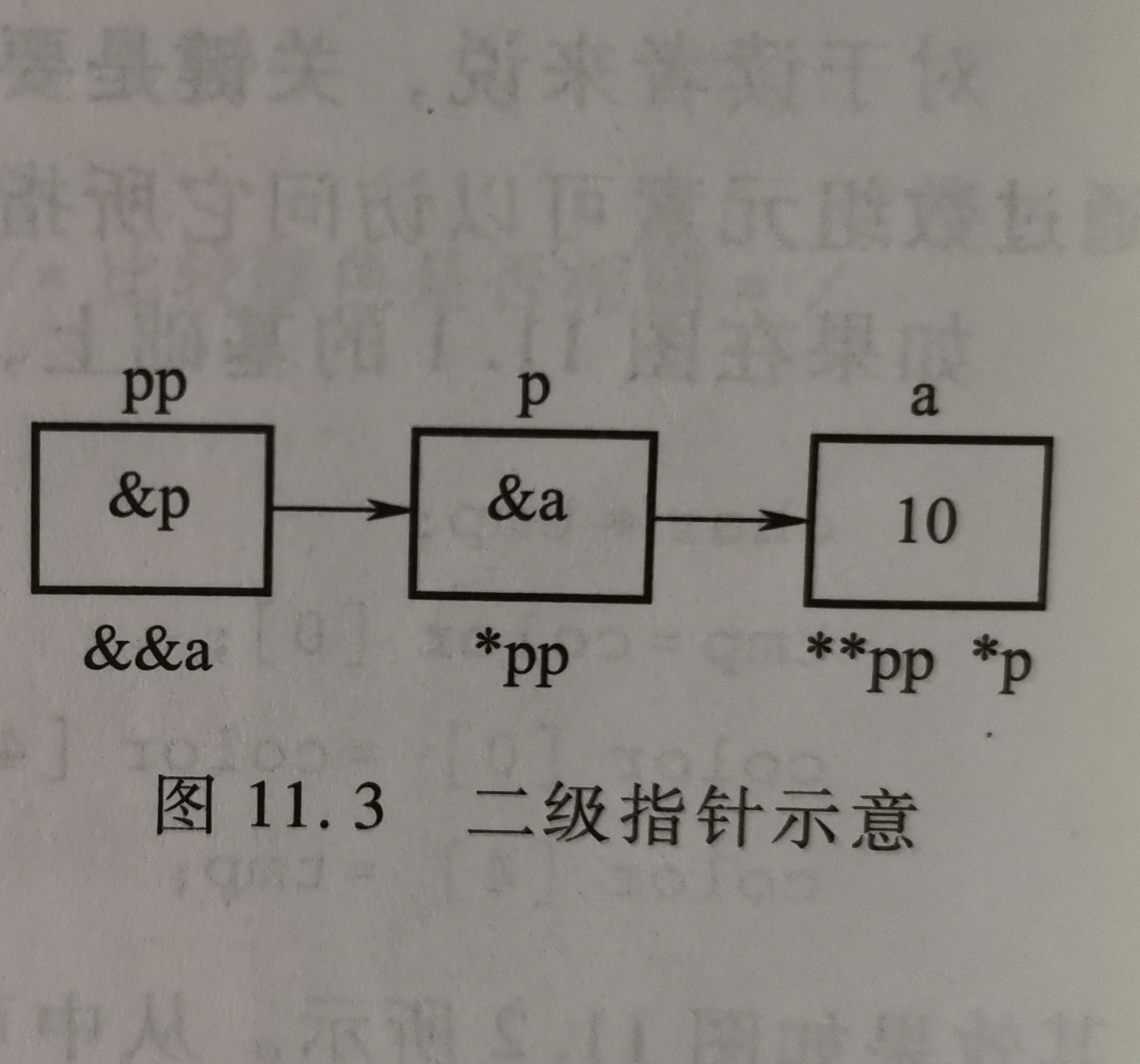## 1.7行指针、列指针

   int a;
int (*pr)=a;//行指针
int *pc=pr;//列指针


1：用列指针输出整个二维数组。
#include <stdio.h>
void main()
{
int a={1,3,5,7,9,11,13,15,17,19,21,23};
int *p= a;   // 列指针的定义法
for(; p < a + 12; p++)
{
printf("%d ",*p);
}
return;
}
2：用行指针输出整个二维数组。
#include <stdio.h>
void main()
{
int a={1,3,5,7,9,11,13,15,17,19,21,23};
int (*p)= &a; // 行指针定义法或者int (*p)= a;
int i, j;
for(i = 0; i < 3; i++)
for(j = 0; j < 4; j++)
{
printf("%d ",*(*(p + i) + j));
}
}


# 2PTA实验作业

## 2.1题目名1

### 2.11伪代码

 for i=0 to 3 then
scanf("%s", word[i]);
len[i] =strlen(word[i]);//长度计算
end for
for i=0 to 3 then
tail[k++] = word[i][len[i] - 2];//倒数第一个字的前半字节
tail[k++] = word[i][len[i] - 1];//倒数第一个数的后半字节
end for
tail[i * 2] = '\0';//加上字符结束标志，为了防止后面出现奇怪的字符


### 2.12代码截图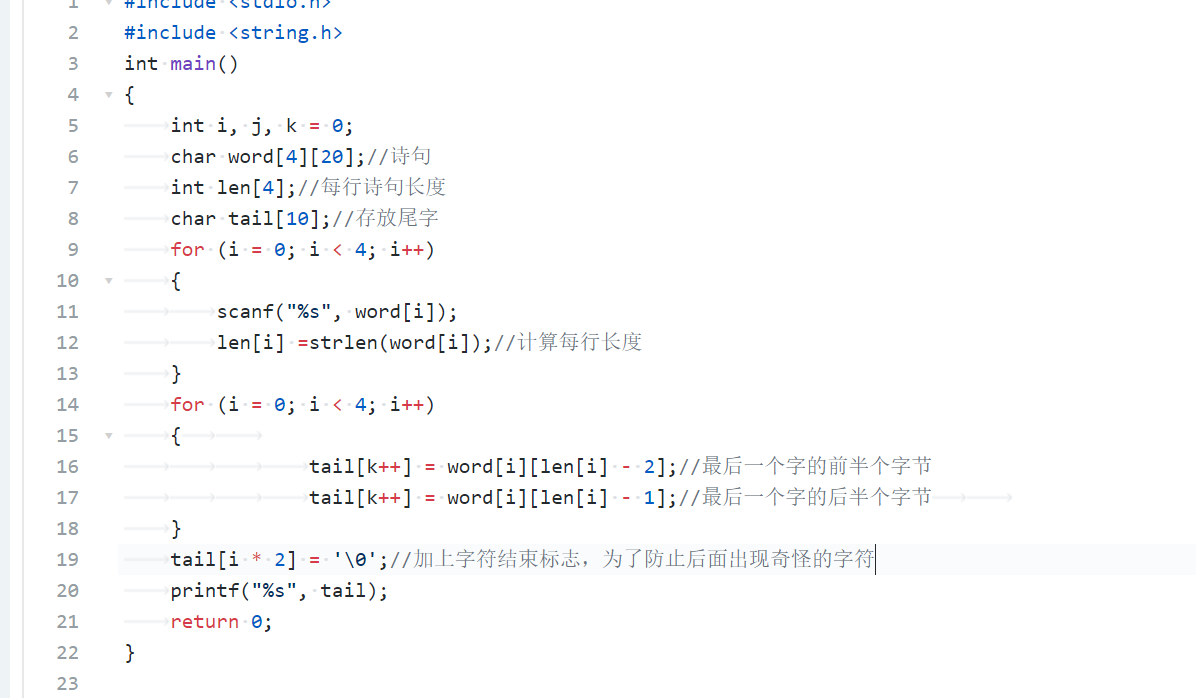### 2.13找一份同学代码（尽量找思路和自己差距较大同学代码）比较，说明各自代码特点。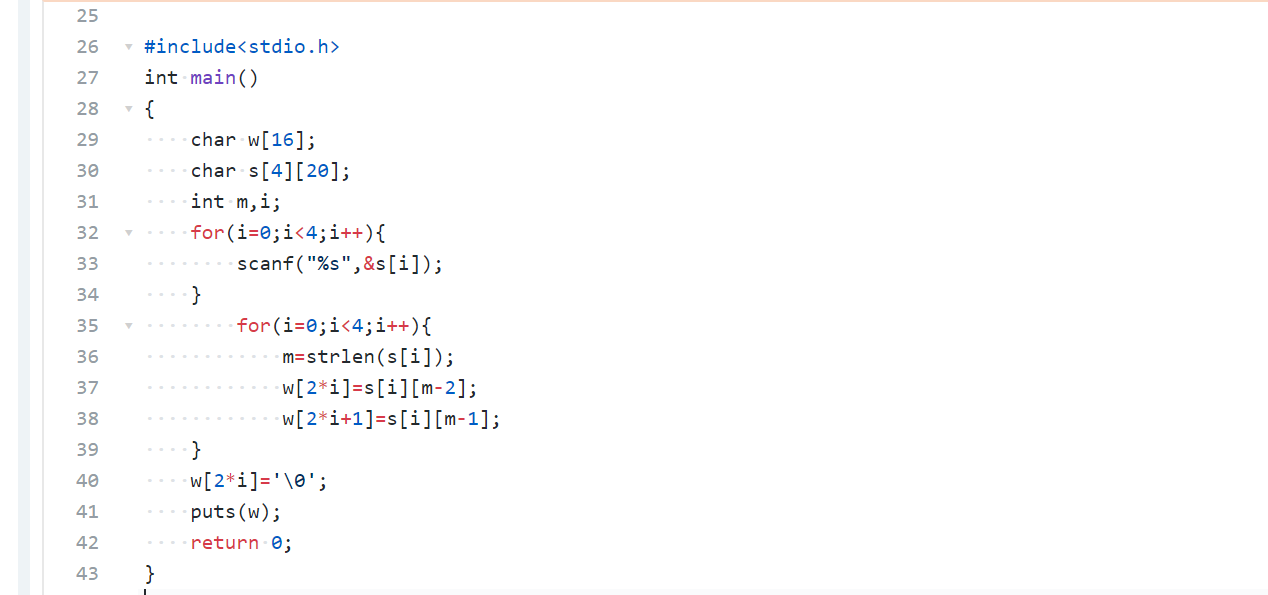## 2.2题目名2“合并2个有序数组”

### 2.21伪代码

     i=m-1;
j=n-1;
k=m+n-1
while i >= 0 && j >= 0
if (a[i] >= b[j])
a[k--] = a[i]
i--
end if
else
a[k--] = b[j]
j--
end else
end while
while j >= 0
a[k--] = b[j]
j--
end while


### 2.22代码截图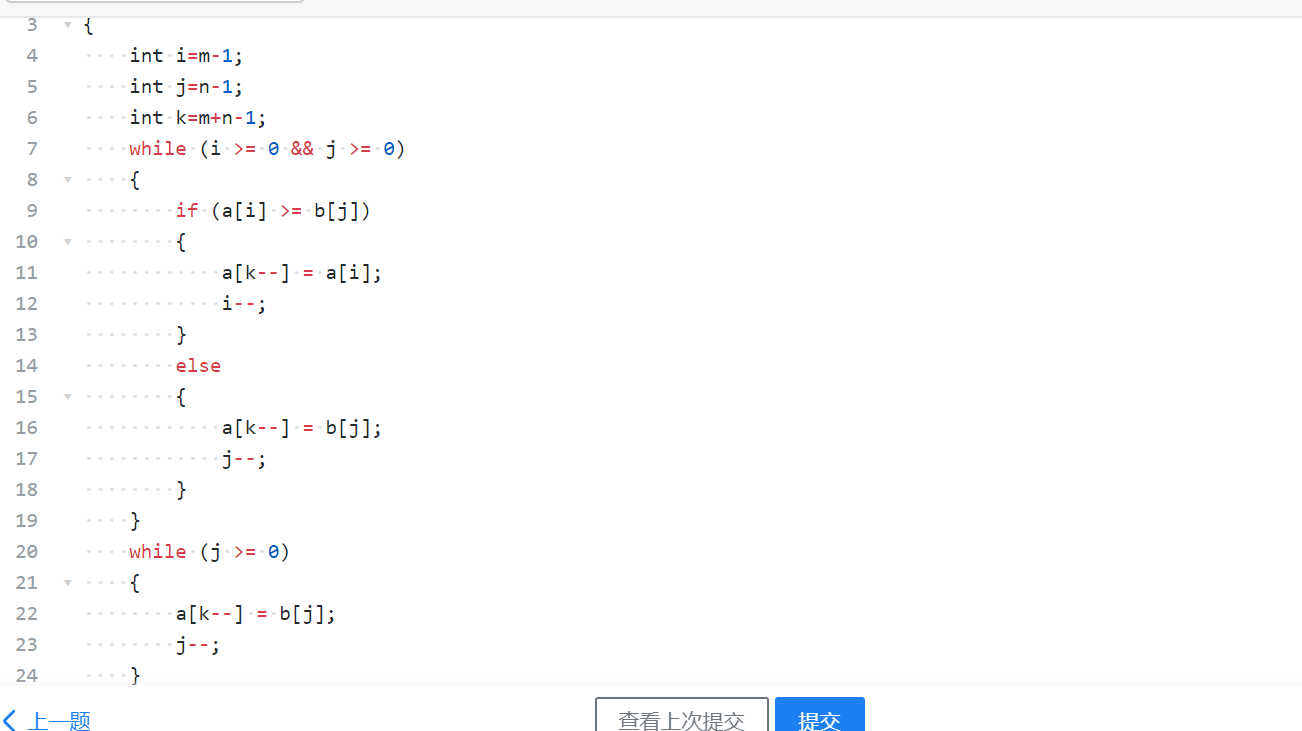### 2.23找一份同学代码（尽量找思路和自己差距较大同学代码）比较，说明各自代码特点。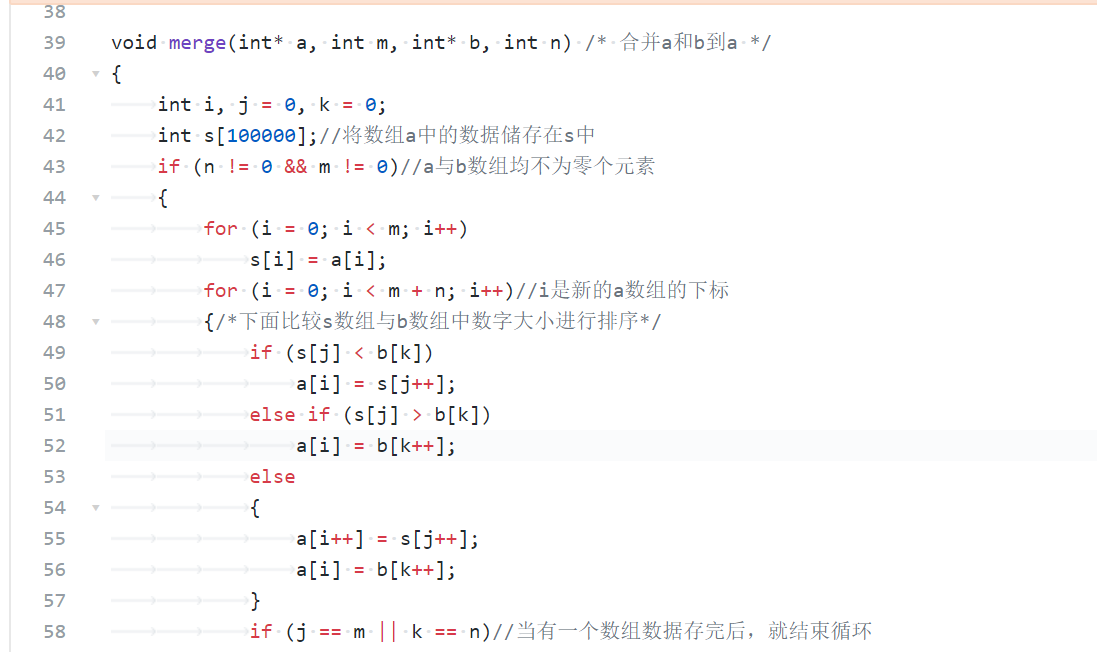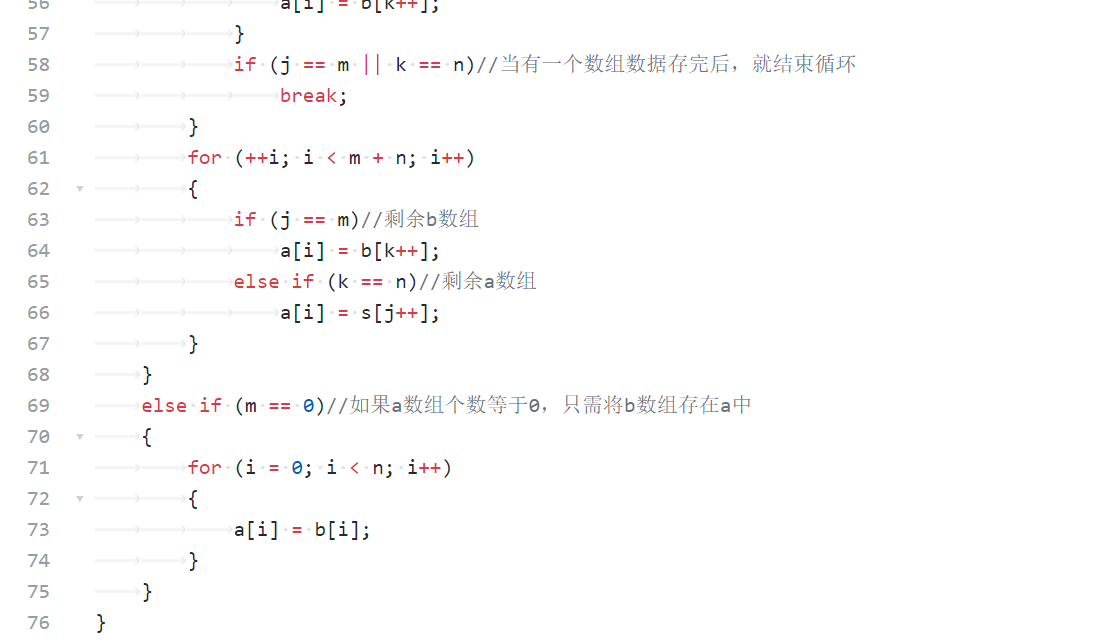## 2.3题目名3“说反话-加强版”

### 2.31伪代码

flag=1
len = strlen(word);//储存字符串长度
len--;
if word[len - 1] == ' '//若最后一个单词后带有空格，将空格改为结束标志'\0'
word[len - 1] = '\0'
flag = 0
end if
len = strlen(word);//更新字符串长度
for i = len - 1 to 0 i--
if word[i] != ' '
last = i;//记录每个单词最后一个字母位置
for j = i - 1 to 0 j--

if word[j] == ' '
break;
end if
end for
temp = j + 1;//记录单词第一个字母位置
if (count > 0)//不是第一次输出的单词，输出单词前需加空格
printf(" ");
end if
for (k = temp; k <= last; k++)
if (flag == 1)//单词结尾不带空格
if (k == len - 1)
continue;//若是要输出原字符串的最后一个单词（不带空格的）
end if
printf("%c", word[k])
end if
end for
count++;//计算输出单词个数
i = j;
end if
end for



### 2.32代码截图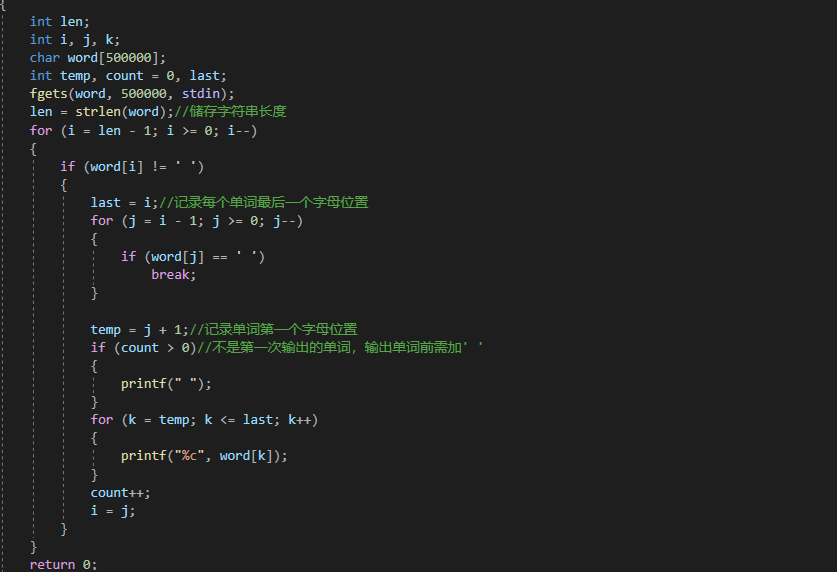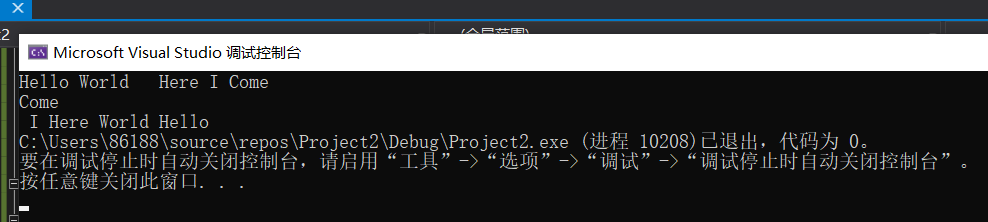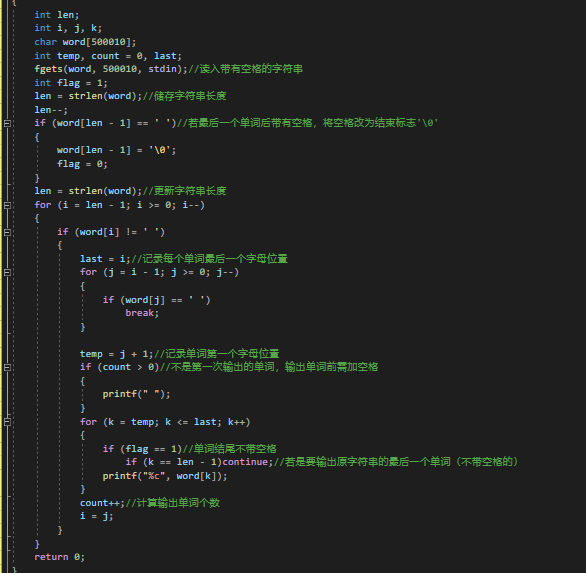### 2.33 请说明和超星视频做法区别，各自优缺点。

posted @ 2020-12-27 21:37  Morri  阅读(119)  评论(0编辑  收藏  举报